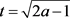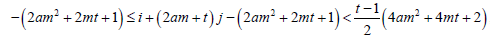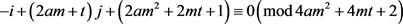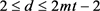﻿ 基于Constacyclic码构造的一类新的量子MDS码

# 基于Constacyclic码构造的一类新的量子MDS码A Class of New Quantum MDS Codes from Constacyclic Codes

Abstract: Quantum MDS codes are an important family of quantum codes. In this paper, we obtain a new class of quantum MDS code of the length n=(q2+1)/aby means of Hermitian construction and constacyclic codes. The result is generalized of the theorem 7 in .

1. 引言

2. 预备知识

2.1. 量子MDS码

2.2. Constacyclic码

$\left(n,q\right)=1$。对于 $\eta \in {F}_{{q}^{2}}^{\text{*}}$，一个长度为n的 ${q}^{2}$ 元类线性码C称为η-constacyclic码当且仅当它在η-constacyclic移位下是不变的：

$\left({c}_{0},{c}_{1},\cdots ,{c}_{n-1}\right)\to \left(\eta {c}_{n-1},{c}_{0},\cdots ,{c}_{n-2}\right)$ .

3. 主要结果

(1) ${C}_{s}=\left\{s\right\}$${C}_{s+n\left(q+1\right)/2}=\left\{s+n\left(q+1\right)/2\right\}$

(2) ${C}_{s-\left(q+1\right)j}=\left\{s-\left(q+1\right)j,s+\left(q+1\right)j\right\},1\le j\le n/2$

$\left[s+n\left(q+1\right)/2\right]{q}^{2}=s{q}^{2}+n\left(q+1\right)\left({q}^{2}-1\right)/2+n\left(q+1\right)/2\equiv s+n\left(q+1\right)/2\left(\mathrm{mod}\left(q+1\right)n\right)$ .

(2) 这个证明类似于  中引理3.12的证明。

(1) 令q是一个素数方幂且 $q=2am+t$，其中a是奇整数，。如果C是一个在 ${F}_{{q}^{2}}$ 上，长度为 $n=\frac{{q}^{2}+1}{a}$ 的η-constacyclic码，并且其定义集合为 $Z={\cup }_{j=0}^{\delta }{C}_{s-\left(q+1\right)j}$，其中 $ord\left(\eta \right)=r$$0\le \delta \le mt$，那么 ${C}^{\perp H}\subseteq C$

(2) 令q是一个素数方幂且 $q=2am-t$，其中a是奇整数， $t=\sqrt{2a-1}$。如果C是一个在 ${F}_{{q}^{2}}$ 上，长度为 $n=\frac{{q}^{2}+1}{a}$ 的η-constacyclic码，并且其定义集合为 $Z={\cup }_{j=0}^{\delta }{C}_{s-\left(q+1\right)j}$，其中 $ord\left(\eta \right)=r$$0\le \delta \le mt-2$，那么 ${C}^{\perp H}\subseteq C$

$i+qj-\frac{{q}^{2}+1}{2}\equiv 0\left(\mathrm{mod}\frac{{q}^{2}+1}{a}\right)$ .

$i+qj-\frac{{q}^{2}+1}{2a}\equiv 0\left(\mathrm{mod}\frac{{q}^{2}+1}{a}\right)$ .

$q=2am+t$，则.

$-\left(2a{m}^{2}+2mt+1\right)\le i+\left(2am+t\right)j-\left(2a{m}^{2}+2mt+1\right)<\frac{t-1}{2}\left(4a{m}^{2}+4mt+2\right)$ ,

$i+\left(2am+t\right)j-\left(2a{m}^{2}+2mt+1\right)=x\left(4a{m}^{2}+4mt+2\right)$，从而 $0\le x\le \frac{t-3}{2}$。因此我们有

$i=2a\left(2x+1\right){m}^{2}+2\left(2x+1\right)mt+2x+1-j\left(2am+t\right)$ .

$-i+qj+\frac{{q}^{2}+1}{2}\equiv 0\left(\mathrm{mod}\frac{{q}^{2}+1}{a}\right)$ .

$-i+qj+\frac{{q}^{2}+1}{2a}\equiv 0\left(\mathrm{mod}\frac{{q}^{2}+1}{a}\right)$ .

$q=2am+t$，则.

$2a{m}^{2}+mt+1\le -i+\left(2am+t\right)j+\left(2a{m}^{2}+2mt+1\right)<\frac{t+1}{2}\left(4a{m}^{2}+4mt+2\right)$ ,

$-i+\left(2am+t\right)j+\left(2a{m}^{2}+2mt+1\right)=x\left(4a{m}^{2}+4mt+2\right)$，从而 $1\le x\le \frac{t-1}{2}$。因此我们有

$i=2a\left(1-2x\right){m}^{2}+2\left(1-2x\right)mt+1-2x+j\left(2am+t\right)$ .

(1) 令q是一个素数方幂且 $q=2am+t$，其中a是奇整数， $t=\sqrt{2a-1}$。那么存在一个参数为 $〚\frac{{q}^{2}+1}{a},\frac{{q}^{2}+1}{a}-2d+2,d〛$ 的q元的量子MDS码，其中 $2\le d\le 2mt+2$ 且d为偶数。

(2) 令q是一个素数方幂且 $q=2am-t$，其中a是奇整数， $t=\sqrt{2a-1}$。那么存在一个参数为 $〚\frac{{q}^{2}+1}{a},\frac{{q}^{2}+1}{a}-2d+2,d〛$ 的q元的量子MDS码，其中且d为偶数。

(1) 令q是一个素数方幂且 $q=10m+3$，那么存在一个参数为 $〚\frac{{q}^{2}+1}{5},\frac{{q}^{2}+1}{5}-2d+2,d〛$ 的q元的量子MDS码，其中 $2\le d\le 6m+2$ 且d为偶数。

(2) 令q是一个素数方幂且 $q=10m-3$，那么存在一个参数为 $〚\frac{{q}^{2}+1}{5},\frac{{q}^{2}+1}{5}-2d+2,d〛$ 的q元的量子MDS码，其中 $2\le d\le 6m-2$ 且d为偶数。

 Calderbank, A.R., Rains, E.M., Shor, P.W. and Sloane, N.J.A. (1998) Quantum Error Correction via Codes over GF(4). IEEE Transactions on Information Theory, 44, 1369-1387.
https://doi.org/10.1109/18.681315

 Ashikhmin, A. and Knill, E. (2001) Nonbinary Quantum Stablizer Codes. IEEE Transactions on Information Theory, 47, 3065-3072.
https://doi.org/10.1109/18.959288

 Chen, H., Ling, S. and Xing, C. (2005) Quantum Codes from Concatenated Algebra-ic-Gemeotric Codes. IEEE Transactions on Information Theory, 51, 2915-2920.
https://doi.org/10.1109/TIT.2005.851760

 Aly, S.A., Klappenecker, A. and Sarvepalli, P.K. (2007) On Quantum and Clas-sical BCH Codes. IEEE Transactions on Information Theory, 53, 1183-1188.
https://doi.org/10.1109/TIT.2006.890730

 Knill, E. and Laflamme, R. (1997) Theory of Quantum Error-Correcting Codes. Physical Review A, 55, 900-911.
https://doi.org/10.1103/PhysRevA.55.900

 Chen, B., Ling, S. and Zhang, G. (2015) Application of Constacyclic Codes to Quantum MDS Codes. IEEE Transactions on Information Theory, 61, 1474-1484.
https://doi.org/10.1109/TIT.2015.2388576

 Li, F. and Yue, Q. (2015) New Quantum MDS-Convolutional Codes Derived from Constacyclic Codes. Modern Physics Letters B, 29, Article ID: 1550252.
https://doi.org/10.1142/S0217984915502528

 Jin, L. and Xing, C. (2014) A Construction of New Quantum MDS Codes. IEEE Transactions on Information Theory, 60, 2921-2925.
https://doi.org/10.1109/TIT.2014.2299800

 Jin, L., Kan, H. and Wen, J. (2017) Quantum MDS Codes with Relatively Large Minimum Distance from Hermitian Self-Orthogonal Codes. Designs Codes and Cryptography, 84, 463-471.
https://doi.org/10.1007/s10623-016-0281-9

 Wang, L. and Zhu, S. (2015) New Quantum MDS Codes Derived from Constacyclic Codes. Quantum Information Processing, 14, 881-889.
https://doi.org/10.1007/s11128-014-0903-y

 Jin, L., Ling, S., Luo, J. and Xing, C. (2010) Application of Classic Hermitian Self-Orthogonal MDS Codes to Quantum MDS Codes. IEEE Transactions on Information Theory, 56, 4735-4740.
https://doi.org/10.1109/TIT.2010.2054174

 Zhang, T. and Ge, G. (2017) Quantum MDS Codes with Large Minimum Dis-tance. Designs Codes and Cryptography 83, 503-517.
https://doi.org/10.1007/s10623-016-0245-0

 Zhang, T. and Ge, G. (2015) Some New Classes of Quantum MDS Codes from Constacyclic Codes. IEEE Transactions on Information Theory, 61, 5224-5228.
https://doi.org/10.1109/TIT.2015.2450235

 Kai, X., Zhu, S. and Li, P. (2014) Constacyclic Codes and Some New Quantum MDS Codes. IEEE Transactions on Information Theory, 60, 2080-2086.
https://doi.org/10.1109/TIT.2014.2308180

 He, X., Xu, L. and Chen, H. (2016) New q-ary Quantum MDS Codes with Distance Bigger than . Quantum Information Processing, 15, 2745-2758.
https://doi.org/10.1007/s11128-016-1311-2

 Shi, X., Yue, Q. and Zhu, X. (2017) Construction of Some New Quantum MDS Codes. Finite Fields and Their Applications, 46, 347-362.
https://doi.org/10.1016/j.ffa.2017.04.002

 Yang, Y. and Cai, W. (2013) On Self-Dual Constacyclic Codes over Finite Fields. Designs, Codes and Cryptography, 74, 355-364.
https://doi.org/10.1007/s10623-013-9865-9

Top